CBSE Class 12 Sample Paper for 2018 Boards

Class 12
Solutions of Sample Papers and Past Year Papers - for Class 12 Boards

### Show that (x − y)dy = (x + 2y)dx is a homogenous differential equation. Also, find the general solution of the given differential equation.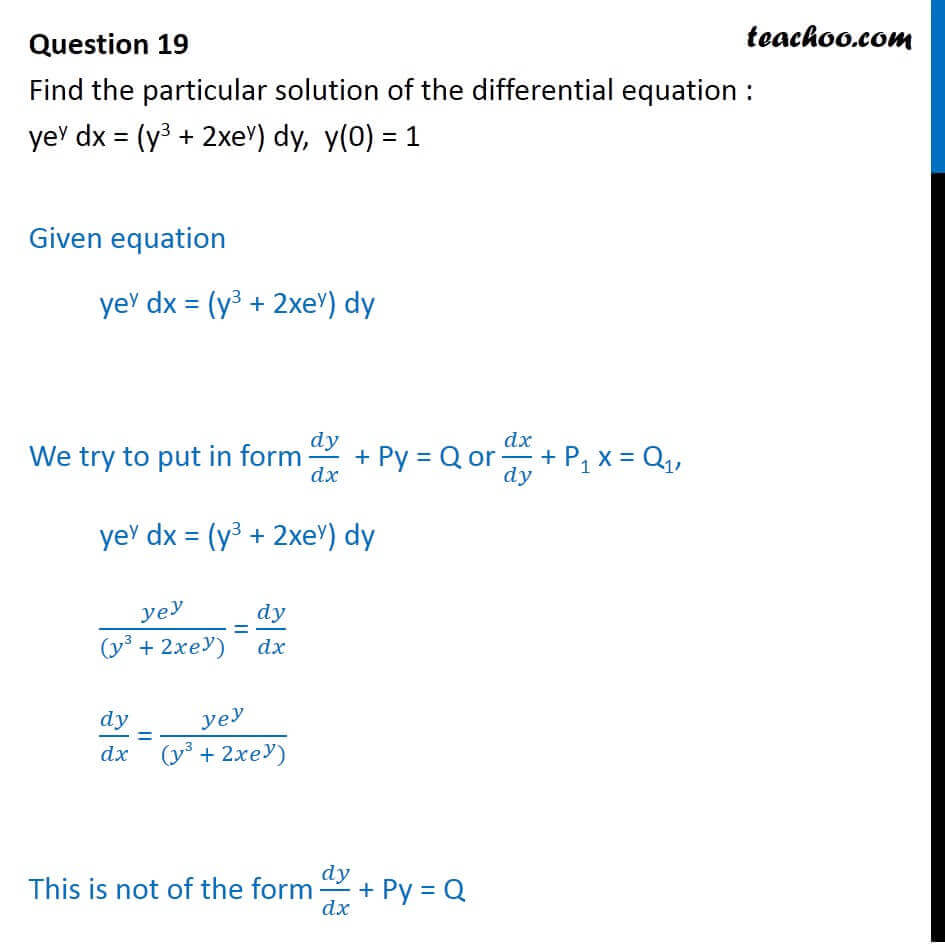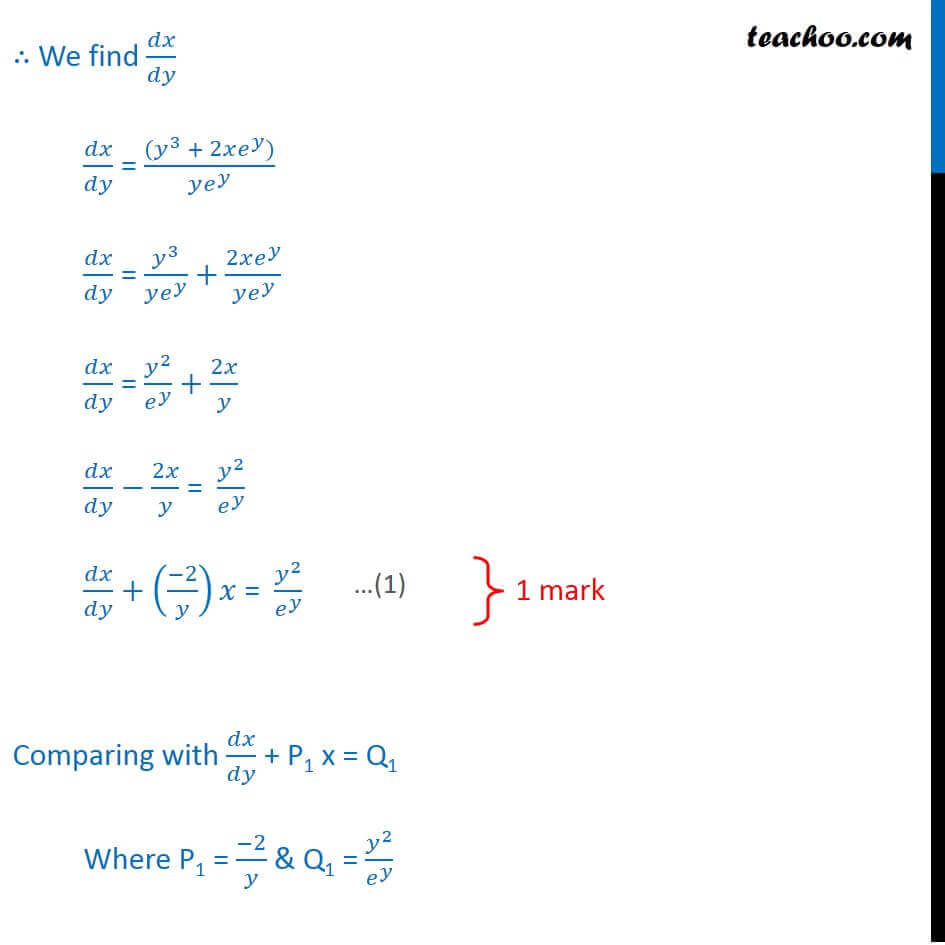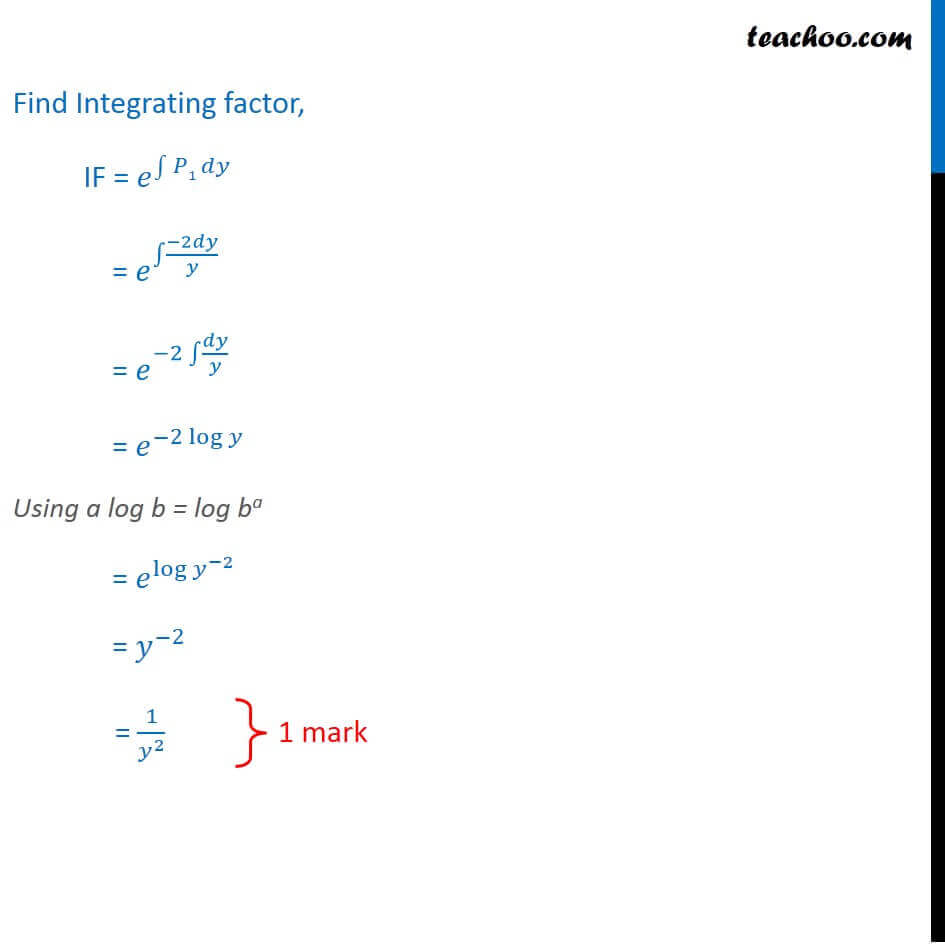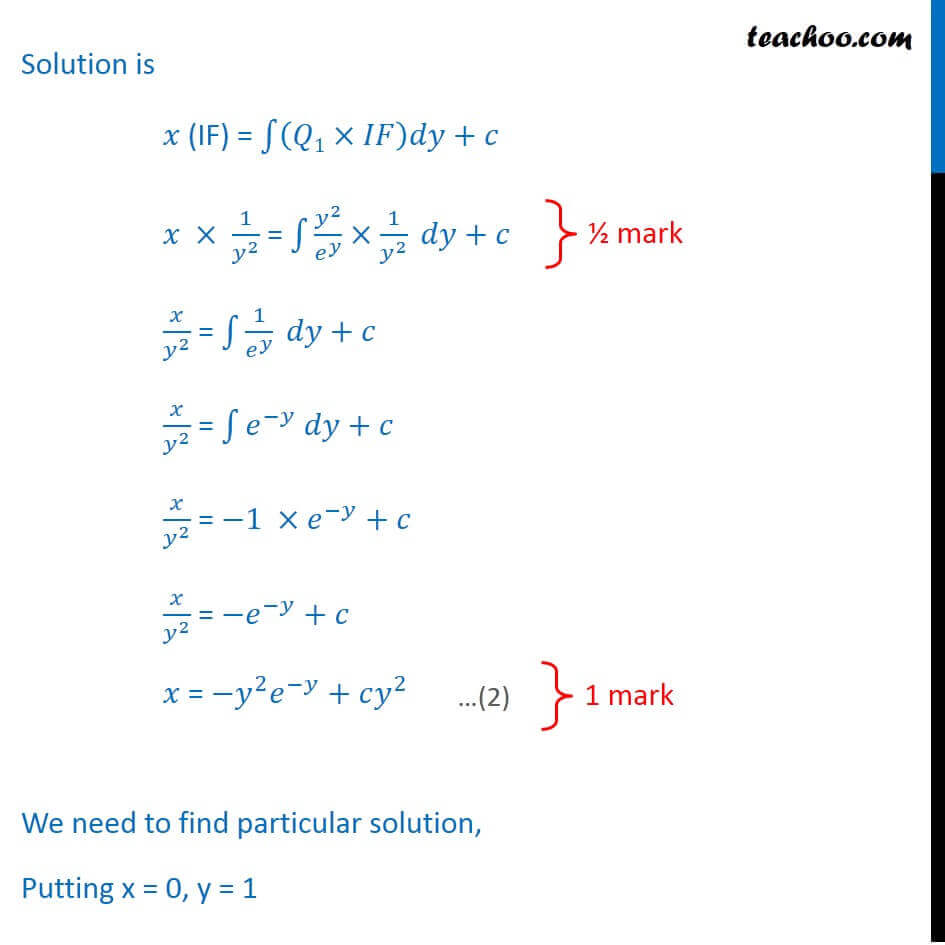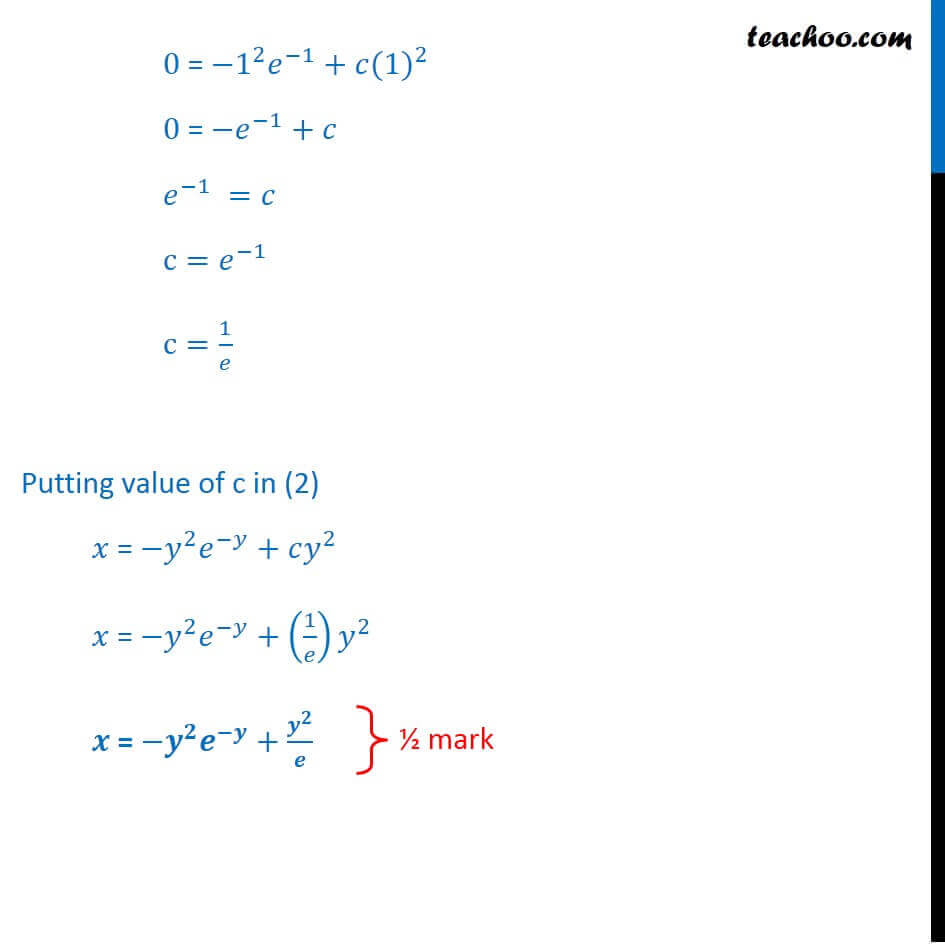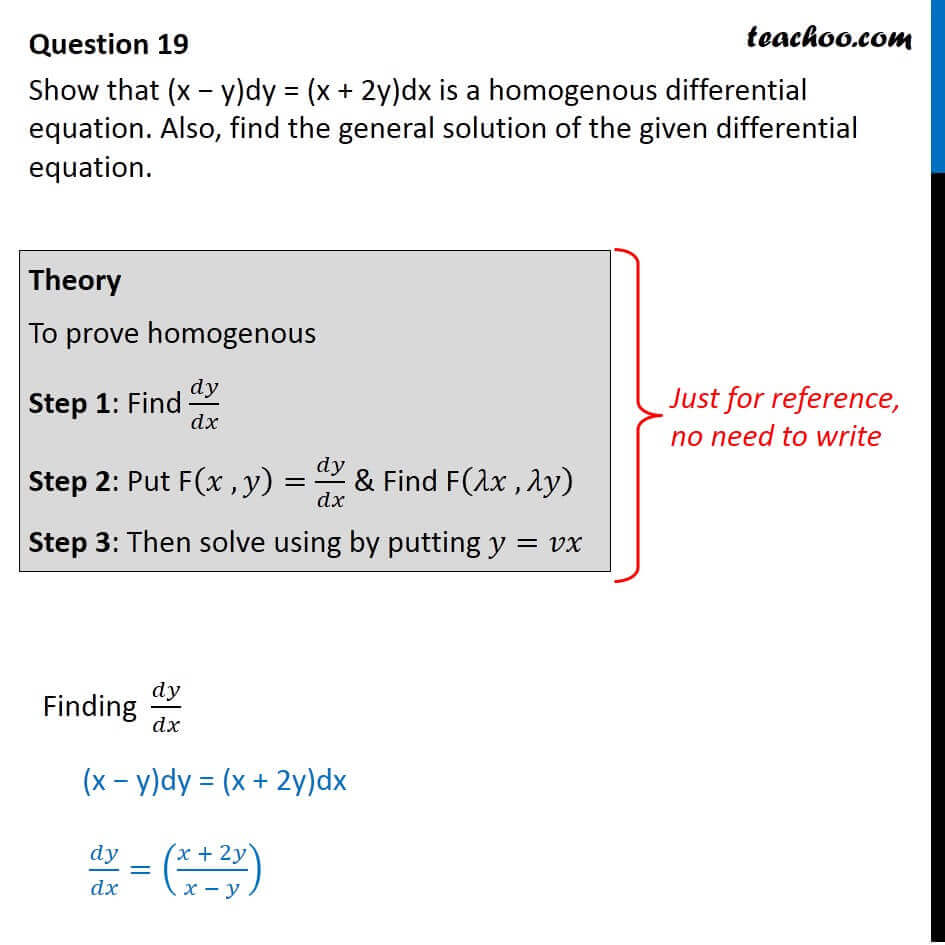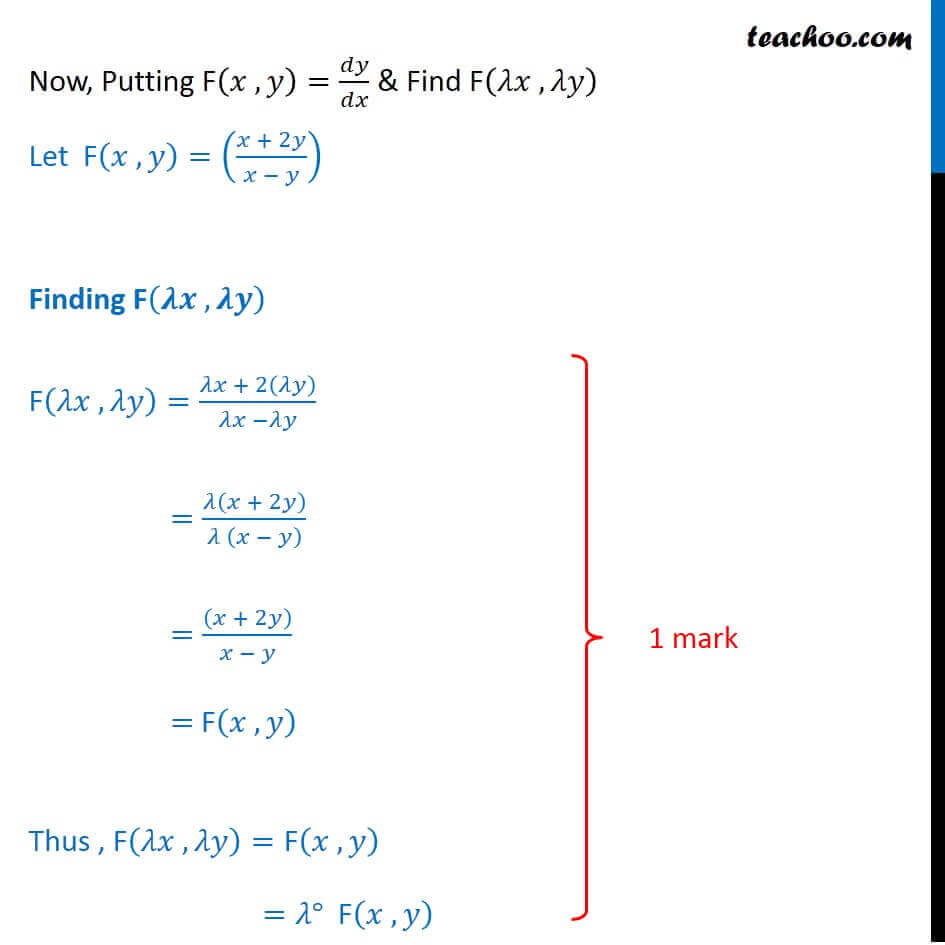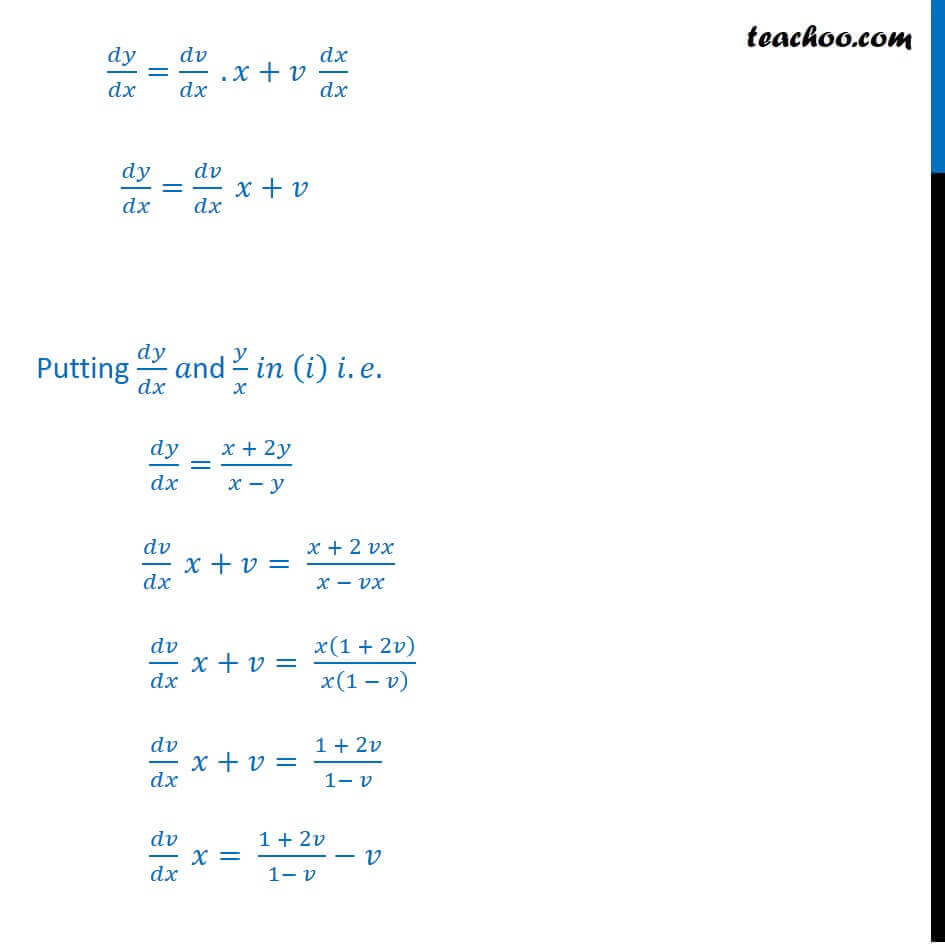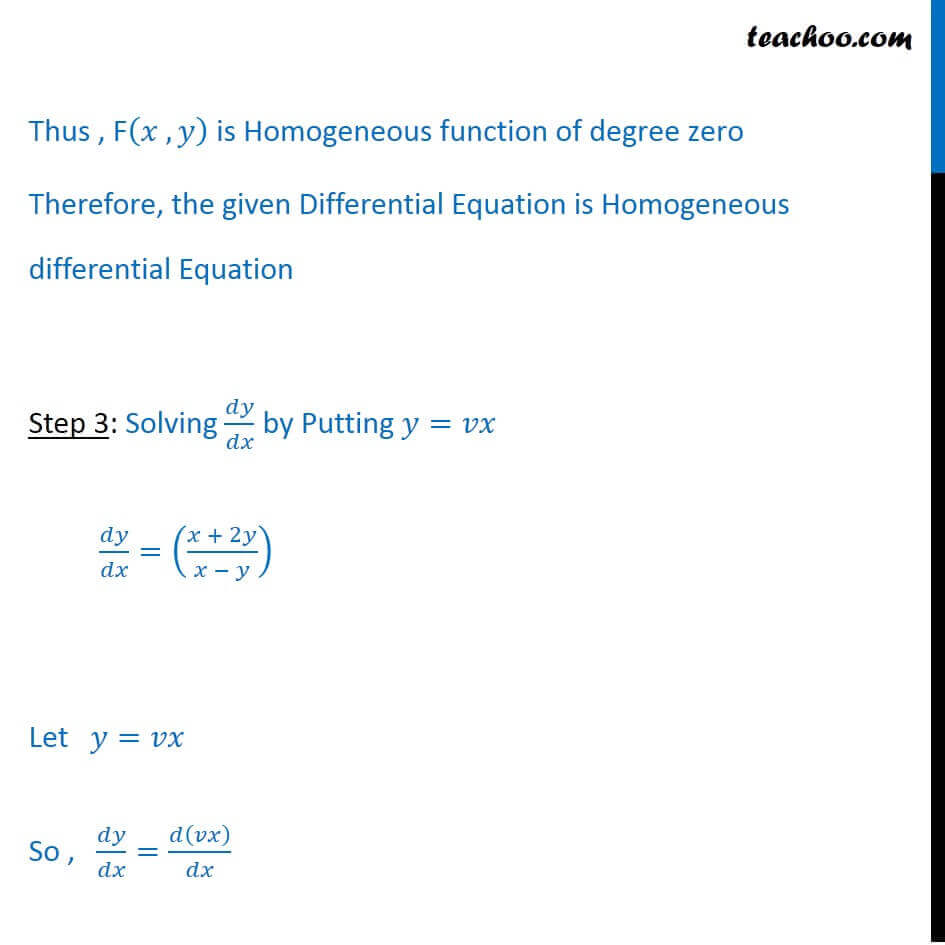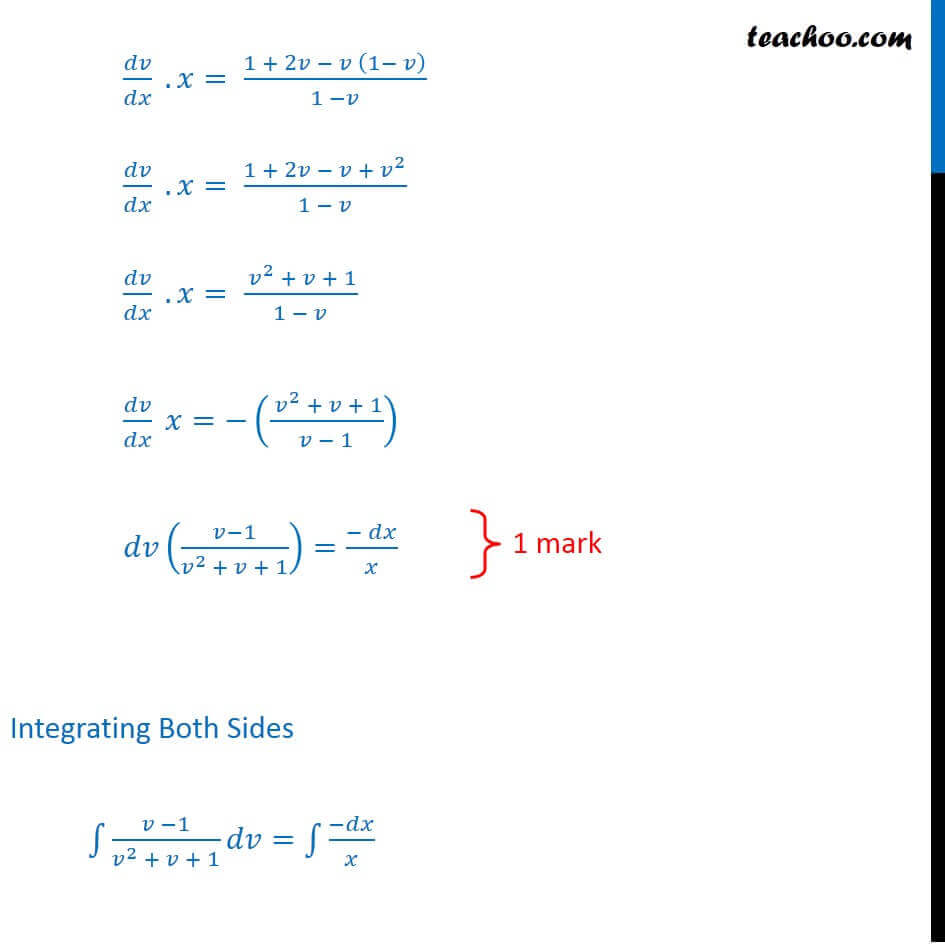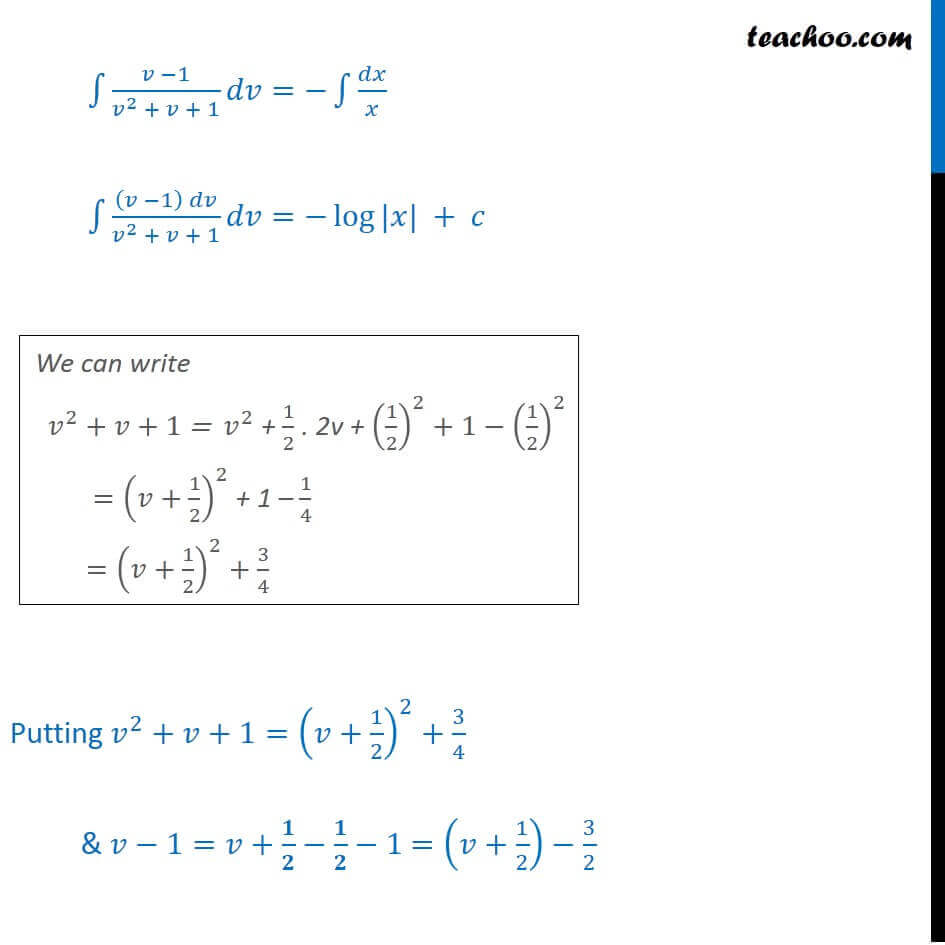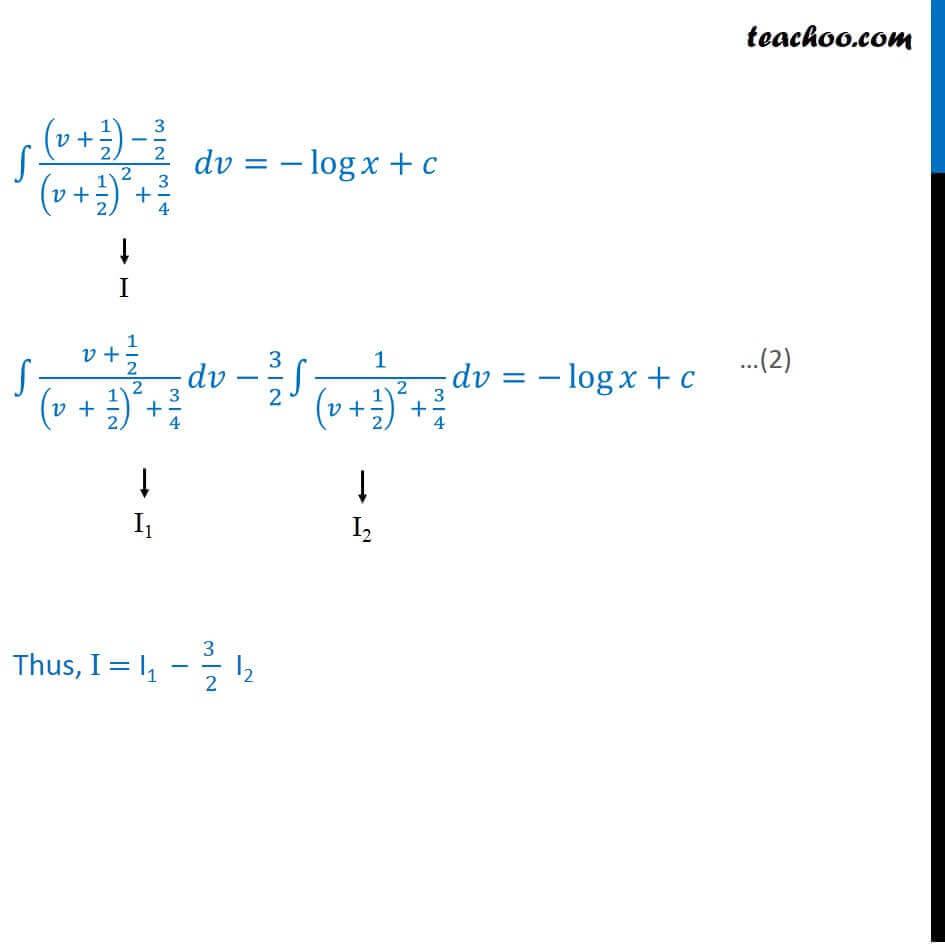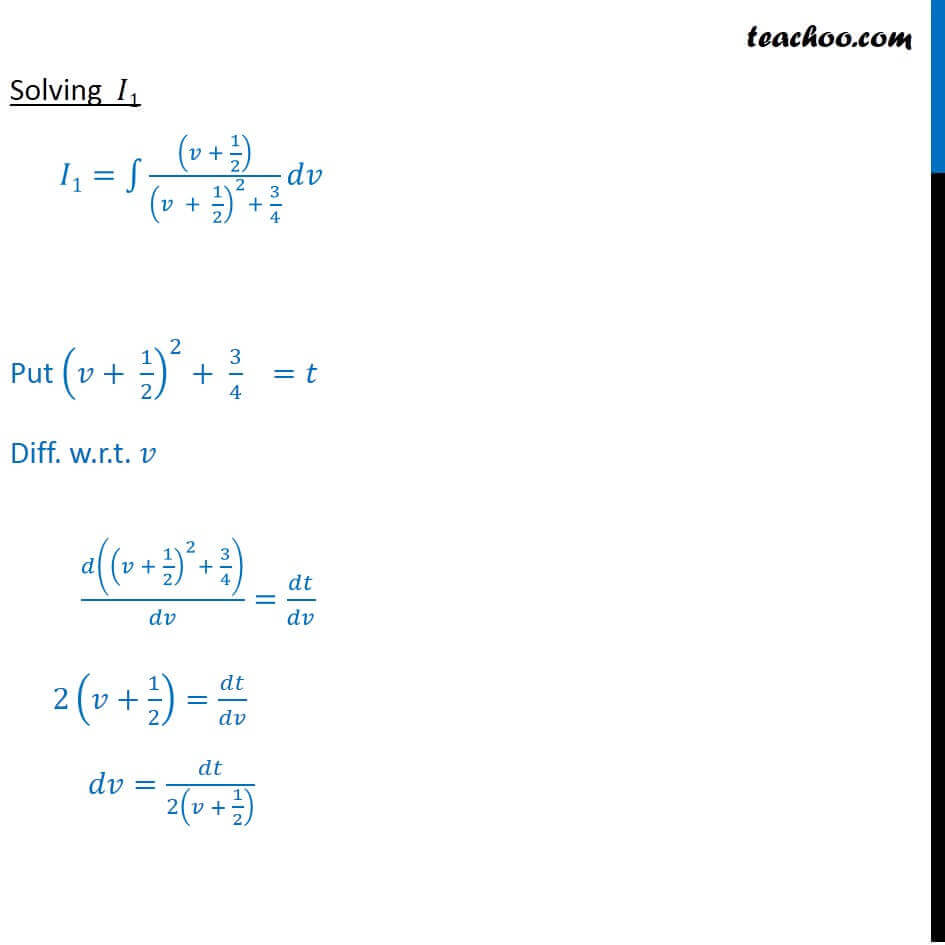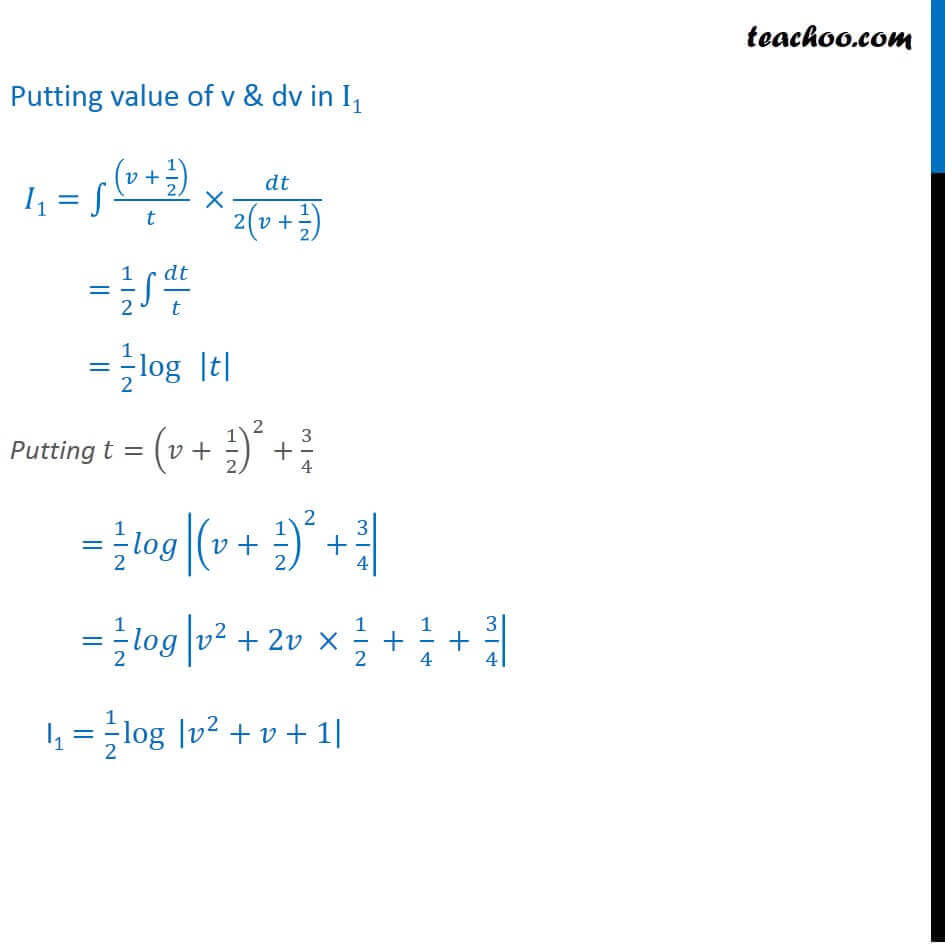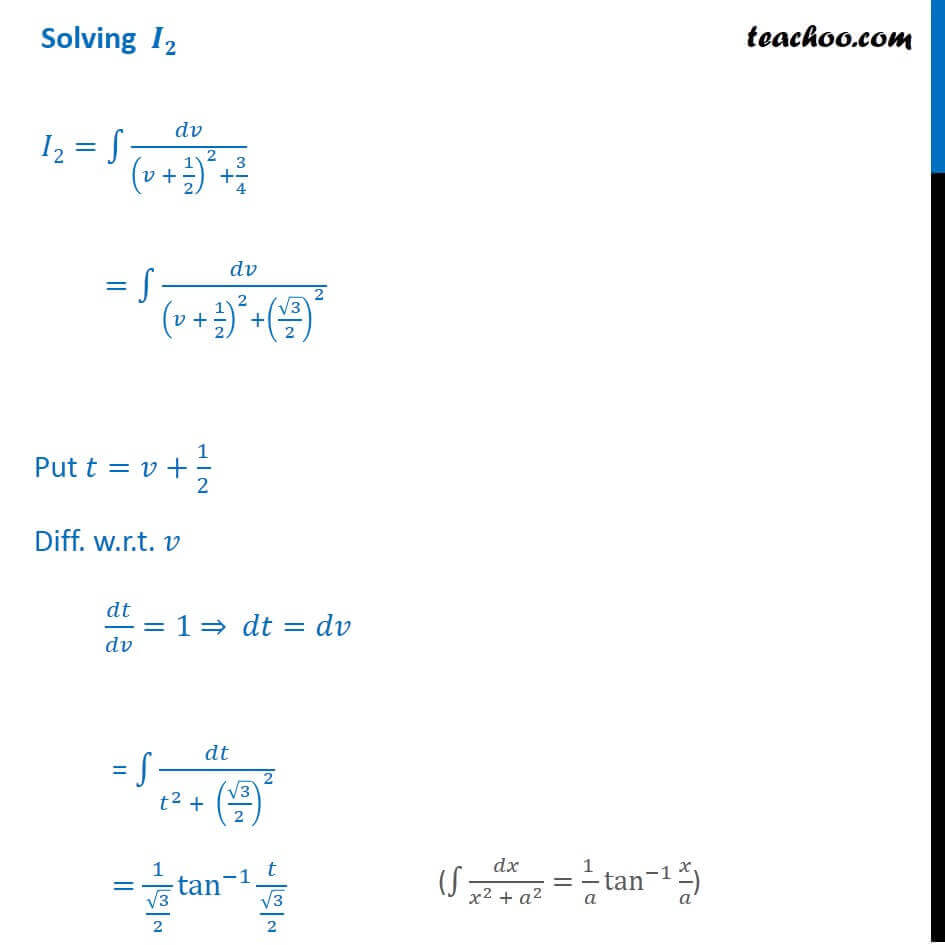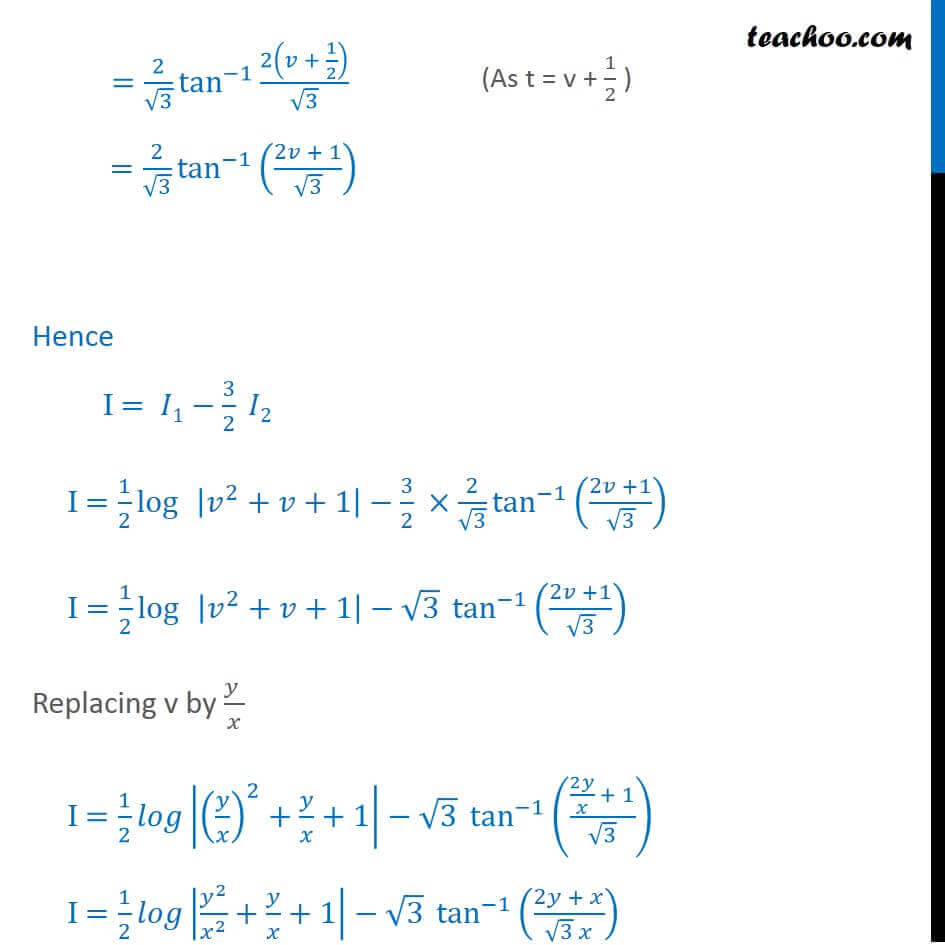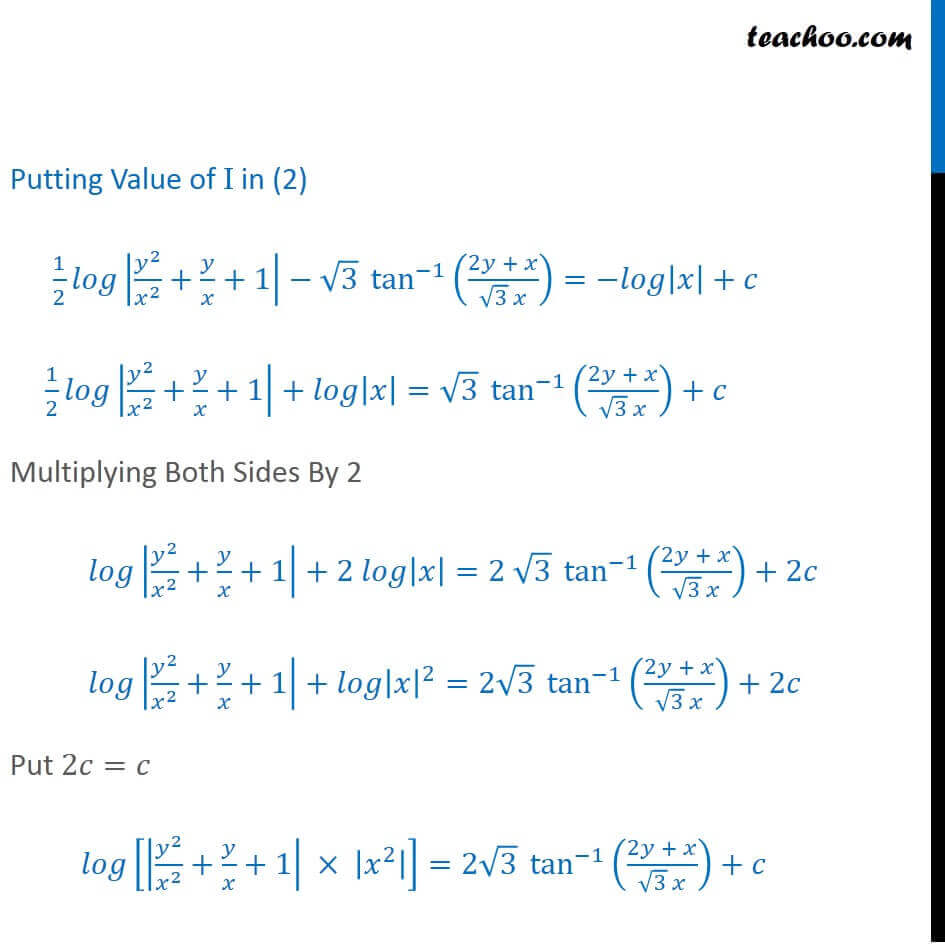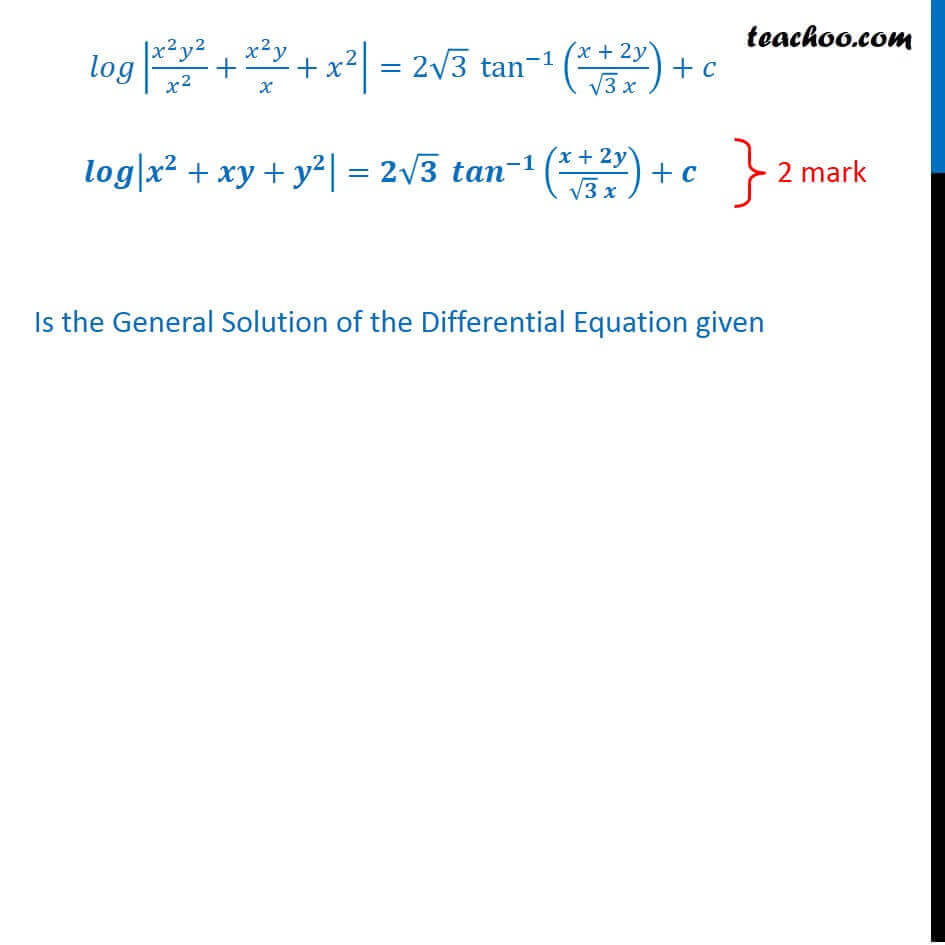### Transcript

Question 19 Find the particular solution of the differential equation : yey dx = (y3 + 2xey) dy, y(0) = 1 Given equation yey dx = (y3 + 2xey) dy We try to put in form 𝑑𝑦/𝑑𝑥 + Py = Q or 𝑑𝑥/𝑑𝑦 + P1 x = Q1, yey dx = (y3 + 2xey) dy (𝑦𝑒^𝑦)/((𝑦3 + 2𝑥𝑒^𝑦)) = 𝑑𝑦/𝑑𝑥 𝑑𝑦/𝑑𝑥 = (𝑦𝑒^𝑦)/((𝑦3 + 2𝑥𝑒^𝑦)) This is not of the form 𝑑𝑦/𝑑𝑥 + Py = Q ∴ We find 𝑑𝑥/𝑑𝑦 𝑑𝑥/𝑑𝑦 = ((𝑦^3 + 2𝑥𝑒^𝑦))/(𝑦𝑒^𝑦 ) 𝑑𝑥/𝑑𝑦 = 𝑦^3/(𝑦𝑒^𝑦 )+(2𝑥𝑒^𝑦)/(𝑦𝑒^𝑦 ) 𝑑𝑥/𝑑𝑦 = 𝑦^2/𝑒^𝑦 +2𝑥/𝑦 𝑑𝑥/𝑑𝑦−2𝑥/𝑦 = 𝑦^2/𝑒^𝑦 𝑑𝑥/𝑑𝑦+((−2)/𝑦)𝑥 = 𝑦^2/𝑒^𝑦 Comparing with 𝑑𝑥/𝑑𝑦 + P1 x = Q1 Where P1 = (−2)/𝑦 & Q1 = 𝑦^2/𝑒^𝑦 Find Integrating factor, IF = 𝑒^∫1▒〖𝑃1 𝑑𝑦〗 = 𝑒^∫1▒(−2𝑑𝑦)/𝑦 = 𝑒^(−2∫1▒𝑑𝑦/𝑦) = 𝑒^(−2 log⁡𝑦 ) Using a log b = log ba = 𝑒^(〖log⁡𝑦〗^(−2) ) = 𝑦^(−2) = 1/𝑦^2 Solution is 𝑥 (IF) = ∫1▒〖(𝑄1×𝐼𝐹)𝑑𝑦+𝑐〗 𝑥 × 1/𝑦^2 = ∫1▒〖𝑦^2/𝑒^𝑦 ×1/𝑦^2 𝑑𝑦+𝑐〗 𝑥/𝑦^2 = ∫1▒〖1/𝑒^𝑦 𝑑𝑦+𝑐〗 𝑥/𝑦^2 = ∫1▒〖𝑒^(−𝑦) 𝑑𝑦+𝑐〗 𝑥/𝑦^2 = −1 ×𝑒^(−𝑦)+𝑐 𝑥/𝑦^2 = 〖−𝑒〗^(−𝑦)+𝑐 𝑥 = 〖−𝑦^2 𝑒〗^(−𝑦)+𝑐𝑦^2 We need to find particular solution, Putting x = 0, y = 1 0 = 〖−1^2 𝑒〗^(−1)+𝑐〖(1)〗^2 0 = 〖−𝑒〗^(−1)+𝑐 𝑒^(−1) =𝑐 c=𝑒^(−1) c=1/𝑒 Putting value of c in (2) 𝑥 = 〖−𝑦^2 𝑒〗^(−𝑦)+𝑐𝑦^2 𝑥 = 〖−𝑦^2 𝑒〗^(−𝑦)+(1/𝑒) 𝑦^2 𝒙 = 〖−𝒚^𝟐 𝒆〗^(−𝒚)+𝒚^𝟐/𝒆 Question 19 Show that (x − y)dy = (x + 2y)dx is a homogenous differential equation. Also, find the general solution of the given differential equation. Theory To prove homogenous Step 1: Find 𝑑𝑦/𝑑𝑥 Step 2: Put F(𝑥 , 𝑦)=𝑑𝑦/𝑑𝑥 & Find F(𝜆𝑥 ,𝜆𝑦) Step 3: Then solve using by putting 𝑦=𝑣𝑥 Finding 𝑑𝑦/𝑑𝑥 (x − y)dy = (x + 2y)dx 𝑑𝑦/𝑑𝑥=((𝑥 + 2𝑦)/(𝑥 − 𝑦)) Now, Putting F(𝑥 , 𝑦)=𝑑𝑦/𝑑𝑥 & Find F(𝜆𝑥 ,𝜆𝑦) Let F(𝑥 , 𝑦)=((𝑥 + 2𝑦)/(𝑥 − 𝑦)) Finding F(𝝀𝒙 ,𝝀𝒚) F(𝜆𝑥 ,𝜆𝑦)=(𝜆𝑥 + 2(𝜆𝑦))/(𝜆𝑥 −𝜆𝑦) =𝜆(𝑥 + 2𝑦)/(𝜆 (𝑥 − 𝑦) ) =((𝑥 + 2𝑦))/(𝑥 − 𝑦) = F(𝑥 , 𝑦) Thus , F(𝜆𝑥 ,𝜆𝑦)="F" (𝑥 , 𝑦)" " =𝜆°" F" (𝑥 , 𝑦)" " Thus , "F" (𝑥 , 𝑦)" is Homogeneous function of degree zero" Therefore, the given Differential Equation is Homogeneous differential Equation Step 3: Solving 𝑑𝑦/𝑑𝑥 by Putting 𝑦=𝑣𝑥 𝑑𝑦/𝑑𝑥=((𝑥 + 2𝑦)/(𝑥 − 𝑦)) Let 𝑦=𝑣𝑥 So , 𝑑𝑦/𝑑𝑥=𝑑(𝑣𝑥)/𝑑𝑥 𝑑𝑦/𝑑𝑥=𝑑𝑣/𝑑𝑥 . 𝑥+𝑣 𝑑𝑥/𝑑𝑥 𝑑𝑦/𝑑𝑥=𝑑𝑣/𝑑𝑥 𝑥+𝑣 Putting 𝑑𝑦/𝑑𝑥 𝑎nd 𝑦/𝑥 𝑖𝑛 (𝑖) 𝑖.𝑒. 𝑑𝑦/𝑑𝑥=(𝑥 + 2𝑦)/(𝑥 − 𝑦) 𝑑𝑣/𝑑𝑥 𝑥+𝑣= (𝑥 + 2 𝑣𝑥)/(𝑥 − 𝑣𝑥) 𝑑𝑣/𝑑𝑥 𝑥+𝑣= 𝑥(1 + 2𝑣)/𝑥(1 − 𝑣) 𝑑𝑣/𝑑𝑥 𝑥+𝑣= (1 + 2𝑣)/(1− 𝑣) 𝑑𝑣/𝑑𝑥 𝑥= (1 + 2𝑣)/(1− 𝑣)−𝑣 𝑑𝑣/𝑑𝑥 . 𝑥= (1 + 2𝑣 − 𝑣 (1− 𝑣))/(1 −𝑣) 𝑑𝑣/𝑑𝑥 . 𝑥= (1 + 2𝑣 − 𝑣 +〖 𝑣〗^2)/(1 − 𝑣) 𝑑𝑣/𝑑𝑥 . 𝑥= (〖 𝑣〗^2 + 𝑣 + 1)/(1 − 𝑣) 𝑑𝑣/𝑑𝑥 𝑥=−((〖 𝑣〗^2 + 𝑣 + 1)/(𝑣 − 1)) 𝑑𝑣((𝑣−1)/(𝑣^(2 )+ 𝑣 + 1))=(− 𝑑𝑥)/𝑥 Integrating Both Sides ∫1▒〖(𝑣 −1)/(𝑣^2 + 𝑣 + 1) 𝑑𝑣=∫1▒(−𝑑𝑥)/𝑥〗 ∫1▒〖(𝑣 −1)/(𝑣^2 + 𝑣 + 1) 𝑑𝑣=−∫1▒𝑑𝑥/𝑥〗 ∫1▒〖((𝑣 −1) 𝑑𝑣)/(𝑣^2 + 𝑣 + 1) 𝑑𝑣〗=−log⁡〖|𝑥|〗 + 𝑐 We can write 𝑣^2+𝑣+1 = 𝑣^2 + 1/2 . 2v + (1/2)^2+1−(1/2)^2 =(𝑣+1/2)^2 + 1 – 1/4 =(𝑣+1/2)^2+3/4 Putting 𝑣^2+𝑣+1=(𝑣+1/2)^2+3/4 & 𝑣−1=𝑣+𝟏/𝟐−𝟏/𝟐−1 =(𝑣+1/2)−3/2 ∫1▒((𝑣 + 1/2) − 3/2)/((𝑣 + 1/2)^2+ 3/4) 𝑑𝑣=−log⁡〖𝑥+𝑐〗 ∫1▒(𝑣 + 1/2)/((𝑣 + 1/2)^2+ 3/4) 𝑑𝑣−3/2 ∫1▒1/((𝑣 + 1/2)^2+ 3/4) 𝑑𝑣=−log⁡〖𝑥+𝑐〗 Thus, I = I1 – (3 )/2 I2 Solving 𝐼1 𝐼1=∫1▒((𝑣 + 1/2))/((𝑣 + 1/2)^2+ 3/4) 𝑑𝑣 Put (𝑣+ 1/2)^2+ 3/4 =𝑡 Diff. w.r.t. 𝑣 𝑑((𝑣 + 1/2)^2+ 3/4)/𝑑𝑣=𝑑𝑡/𝑑𝑣 2(𝑣+1/2)=𝑑𝑡/𝑑𝑣 𝑑𝑣=𝑑𝑡/2(𝑣 + 1/2) Putting value of v & dv in I1 𝐼1=∫1▒((𝑣 + 1/2))/𝑡 ×𝑑𝑡/2(𝑣 + 1/2) =1/2 ∫1▒𝑑𝑡/𝑡 =1/2 log⁡ |𝑡| Putting 𝑡=(𝑣+ 1/2)^2+3/4 =1/2 𝑙𝑜𝑔|(𝑣+ 1/2)^2+3/4| =1/2 𝑙𝑜𝑔|𝑣^2+2𝑣 × 1/2 + 1/4 + 3/4| I1 =1/2 log⁡〖 |𝑣^2+𝑣+1|〗 Solving 𝑰𝟐 𝐼2=∫1▒𝑑𝑣/((𝑣 + 1/2)^2+3/4) =∫1▒𝑑𝑣/((𝑣 + 1/2)^2+(√3/2)^2 ) Put 𝑡=𝑣+1/2 Diff. w.r.t. 𝑣 𝑑𝑡/𝑑𝑣=1 ⇒ 𝑑𝑡=𝑑𝑣 = ∫1▒𝑑𝑡/(𝑡^2 + 〖 (√3/2)〗^2 ) =1/(√3/2) tan^(−1)⁡〖𝑡/(√3/2)〗 =2/√3 tan^(−1)⁡〖2(𝑣 + 1/2)/√3〗 =2/√3 tan^(−1)⁡((2𝑣 + 1)/√3) Hence I = 𝐼1−3/2 𝐼2 I =1/2 log⁡ |𝑣^2+𝑣+1|−3/2 ×2/√3 tan^(−1)⁡((2𝑣 +1)/√3) I =1/2 log⁡ |𝑣^2+𝑣+1|−√3 tan^(−1)⁡((2𝑣 +1)/√3) Replacing v by (𝑦 )/𝑥 I =1/2 𝑙𝑜𝑔|(𝑦/𝑥)^2+𝑦/𝑥+1|−√3 tan^(−1)⁡((2𝑦/𝑥 + 1)/√3) I =1/2 𝑙𝑜𝑔|𝑦^2/𝑥^2 +𝑦/𝑥+1|−√3 tan^(−1)⁡((2𝑦 + 𝑥)/(√3 𝑥)) Putting Value of I in (2) 1/2 𝑙𝑜𝑔|𝑦^2/𝑥^2 +𝑦/𝑥+1|−√3 tan^(−1)⁡((2𝑦 + 𝑥)/(√3 𝑥))=−𝑙𝑜𝑔|𝑥|+𝑐 1/2 𝑙𝑜𝑔|𝑦^2/𝑥^2 +𝑦/𝑥+1|+𝑙𝑜𝑔|𝑥|=√3 tan^(−1)⁡((2𝑦 + 𝑥)/(√3 𝑥))+𝑐 Multiplying Both Sides By 2 𝑙𝑜𝑔|𝑦^2/𝑥^2 +𝑦/𝑥+1|+2 𝑙𝑜𝑔|𝑥|=2 √3 tan^(−1)⁡((2𝑦 + 𝑥)/(√3 𝑥))+2𝑐 𝑙𝑜𝑔|𝑦^2/𝑥^2 +𝑦/𝑥+1|+𝑙𝑜𝑔|𝑥|^2=2√3 tan^(−1)⁡((2𝑦 + 𝑥)/(√3 𝑥))+2𝑐 Put 2𝑐=𝑐 𝑙𝑜𝑔[|𝑦^2/𝑥^2 +𝑦/𝑥+1| × |𝑥^2 |]=2√3 tan^(−1)⁡((2𝑦 + 𝑥)/(√3 𝑥))+𝑐 𝑙𝑜𝑔|〖𝑥^2 𝑦〗^2/𝑥^2 +(𝑥^2 𝑦)/𝑥+𝑥^2 |=2√3 tan^(−1)⁡((𝑥 + 2𝑦)/(√3 𝑥))+𝑐 𝒍𝒐𝒈|𝒙^𝟐+𝒙𝒚+𝒚^𝟐 |=𝟐√𝟑 〖𝒕𝒂𝒏〗^(−𝟏)⁡((𝒙 + 𝟐𝒚)/(√𝟑 𝒙))+𝒄 Is the General Solution of the Differential Equation given